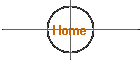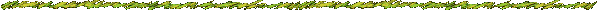Apparent and Absolute Magnitudes

Apparent Magnitude

Apparent magnitude m of a star is a number that tells how bright that star appears at its great distance from Earth. The scale is "backwards" and logarithmic. Larger magnitudes correspond to fainter stars. Note that brightness is another way to say the flux of light, in Watts per square meter, coming towards us.

On this magnitude scale, a brightness ratio of 100 is set to correspond exactly to a magnitude difference of 5. As magnitude is a logarithmic scale, one can always transform a brightness ratio B2/B1 into the equivalent magnitude difference m2-m1 by the formula:

m2-m1 = -2.50 log(B2/B1).

You can check that for brightness ratio B2/B1=100, we have log(B2/B1) =log(100)= log(102) = 2, and then m2-m1=-5, the basic definition of this scale (brighter is more negative m). One then has the following magnitudes and their corresponding relative brightnesses:

magnitude m   |  0   1   2   3   4   5   6   7   8    9    10
----------------------------------------------------------------
relative      |  1  2.5 6.3 16  40  100 250 630 1600 4000 10,000
brightness    |
ratios       |

(Note that the lower row of numbers is just (2.512)m.)Absolute Magnitude

Absolute magnitude Mv is the apparent magnitude the star would have if it were placed at a distance of 10 parsecs from the Earth. Doing this to a star (it is a little difficult), will either make it appear brighter or fainter. From the inverse square law for light, the ratio of its brightness at 10 pc to its brightness at its known distance d (in parsecs) is

B10/Bd=(d/10)2.

Then, like the formula above, we say that its absolute magnitude is

Mv = m - 2.5 log[ (d/10)2 ].

Stars farther than 10 pc have Mv more negative than m, that is why there is a minus sign in the formula. If you use this formula, make sure you put the star's distance d in parsecs (1 pc = 3.26 ly = 206265 AU).Distance Determination

The above relation can also be used to determine the distance to a star if you know both its apparent magnitude and absolute magnitude. This would be the case, for example, when one uses Cepheid or other variable stars for distance determination. Turning the formula inside out:

d = (10 pc) x 10(m-Mv)/5

For example, for a Cepheid variable with Mv = -4, and m = 18, the distance is

d = (10 pc) x 10[18-(-4)]/5 = 2.51 x 105 pc.

THank you to John Murrell for recommending a correction to the previous page. (5/11/2017)
Credit to:  wysin@phys.ksu.edu